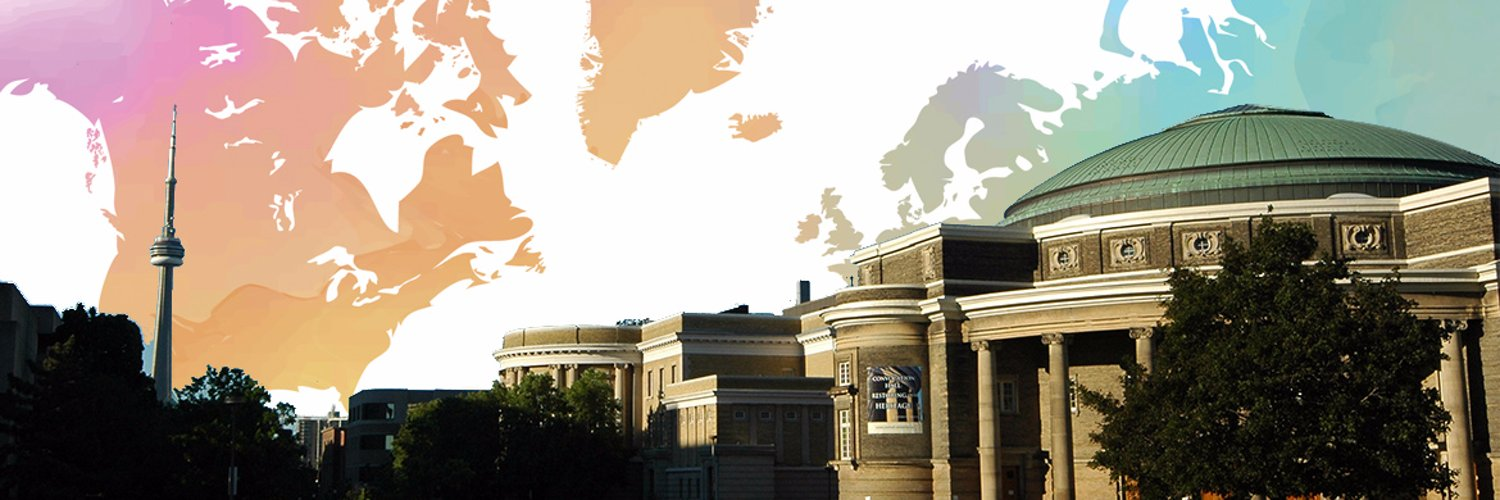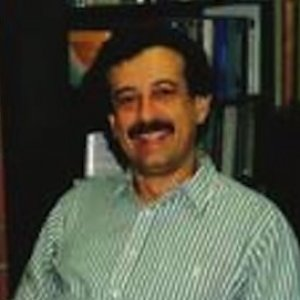# William Weiss

Professor and Chief | University of Toronto, Department of Mathematics

William Weiss is a mathematics professor at University Of Toronto St George Campus.

## Articles (4)

#### Ergodicity of Markov processes via non-standard analysis

Probability

2016 A time-homogeneous Markov process with a stationary probability distribution π will converge to π in an appropriate sense (i.e., will be “ergodic”), under suitable conditions (such as “irreducibility”). This phenomenon is well understood for processes in discrete time and space, and for processes in continuous time and discrete space (see e.g. ), and for processes in discrete time and continuous space. However, for processes in continuous time and space, there are apparently no such clean results; the closest are apparently the results in using awkward assumptions about skeleton chains (see Section 5 below)...

view more

arXiv

2015

view more

#### Variations on ω-boundedness

Israel Journal of Mathematics

2013 Let be a property (or, equivalently, a class) of topological spaces. A space X is called-bounded if every subspace of X with (or in) has compact closure. Thus, countable-bounded has been known as ω-bounded and (σ-compact)-bounded as strongly ω- ...

view more

#### Universal functions

arXiv

2012 A function of two variables F (x, y) is universal iff for every other function G (x, y) there exists functions h (x) and k (y) with G (x, y)= F (h (x), k (y)) Sierpinski showed that assuming the continuum hypothesis there exists a Borel function F (x, y) which is universal ...

view more